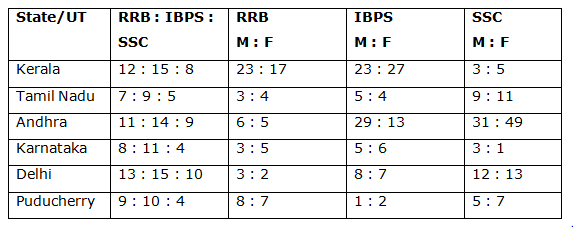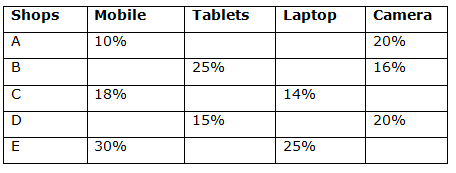# Quantitative Aptitude Questions (Data Interpretation) for RRB PO Mains 2018 Day-176

Dear Readers, IBPS RRB is conducting Online Examination for the recruitment of Assistant. To enrich your preparation here we have providing new series of Data Interpretation – Quantitative Aptitude Questions. Candidates those who are appearing in RRB PO Mains Exams can practice these Quantitative Aptitude average questions daily and make your preparation effective.

[WpProQuiz 3356]

Click “Start Quiz” to attend these Questions and view Solutions

Directions (1 – 5): Study the following information carefully and answer the given questions.

The following table shows the ratio of candidates passed in different competitive examinations and the ratio of male and female among them.1) If the total number of candidates who passed from Karnataka in IBPS is 440, then the total number of candidates who passed from Karnataka is approximately what percentage of total number of male candidates who passed from Karnataka in SSC?

a) 520 %

b) 630 %

c) 767 %

d) 445 %

e) 325 %

2) If the total number of females who passed from Delhi in IBPS is 175, then find the total number of males who passed from Delhi in SSC?

a) 120

b) 270

c) 180

d) 230

e) 90

3) If the total number of candidates passed from Puducherry in all the given three competitive examination is 345, then find the ratio between the total number of male candidates passed in RRB to that of total number of female candidates passed in IBPS from Puducherry?

a) 11 : 17

b) 5 : 13

c) 23 : 35

d) 18 : 25

e) None of these

4) Total number of candidates passed from Kerala in RRB is same as the total number of candidates passed from Tamil Nadu in IBPS, which is equal to 360. Find the difference between the total number of candidates passed from Kerala in SSC to that of total number of candidates passed from Tamil Nadu in RRB?

a) 80

b) 40

c) 120

d) 100

e) 60

5) If the total number of candidates passed from Andhra in IBPS is 420, then find the average number of candidates passed from Andhra in RRB, IBPS and RRB?

a) 340

b) 375

c) 250

d) 220

e) None of these

Directions (6 – 10): Study the following information carefully and answer the given questions.

The following table shows the percentage of discount allowed by different shops on different items.6) The marked price of Mobile sold by shop C and E were equal and the total discount allowed by these shops was Rs. 9600 then find the cost price of the mobile sold by shop C if the shopkeeper earned 25% profit on the mobile?

a) Rs. 13120

b) Rs. 15300

c) Rs. 18250

d) Rs. 20750

e) None of these

7) The selling price of laptop sold by shop D was equal to the total selling price of tablet and camera sold by the same shop while the difference between the selling price of tablet and camera is Rs. 1500 and both the items (tablet and camera) have same market price, then find the profit earned in laptop sold by the shopkeeper of shop D if he earns profit of 10% by selling the laptop?

a) Rs. 5300

b) Rs. 4500

c) Rs. 3000

d) Rs. 2000

e) Rs. 1600

8) The selling price of mobile and tablet of shop B is in ratio of 2: 3. If the shopkeeper earns a profit of 25% on mobile which is equal to Rs. 500 and 20% on tablet then find the total profit earned by the seller?

a) 1125

b) 1120

c) 3015

d) 2023

e) 1612

9) If the selling price of camera sold by shop A is Rs. 28000 and the profit earned by the shopkeeper is 25% then find the difference between the profits earned by the seller if he decreases the rate of discount to 10% from 20%?

a) Rs. 2800

b) Rs. 1500

c) Rs. 3500

d) Rs. 2400

e) None of these

10) If the marked price of laptop sold by shop E is twice the camera sold by shop B and total selling price of these items is Rs. 18720 then find the profit earned by shop E on selling the laptop if percentage profit on the laptop is 20%?

a) Rs. 1300

b) Rs. 1500

c) Rs. 3000

d) Rs. 2000

e) None of these

Direction (1-5) :

The total number of candidates who passed from Karnataka in IBPS = 440

The ratio of total number of candidates passed from Karnataka in RRB, IBPS and SSC = 8 : 11 : 4

11’s = 440

1’s = 40

Total candidates = 23’s = 920

Total number of candidates who passed from Karnataka in SSC

= > 4*40 = 160

Total number of male candidates who passed from Karnataka in SSC

= > 160*(3/4) = 120

Required % = (920/120)*100 = 767 %

The total number of females who passed from Delhi in IBPS = 175

= > 7’s = 175

= > 1’s = 25

The total number of candidates who passed from Delhi in IBPS

= > 15’s = 15*25 = 375

The total number of candidates who passed from Delhi in SSC

= > (375/15)*10 = 250

The total number of males who passed from Delhi in SSC

= > 250*(12/25) = 120

The total number of candidates passed from Puducherry in all the given three competitive examination = 345

The ratio of candidates passed from Puducherry in RRB, IBPS and SSC

= > 9: 10: 4

23’s = 345

1’s = 345/23 = 15

The total number of male candidates passed in RRB from Puducherry

= > (15*9)*(8/15) = 72

The total number of female candidates passed in IBPS from Puducherry

= > (15*10)*(2/3) = 100

Respective ratio = 72: 100 = 18: 25

Total number of candidates passed from Kerala in RRB = 360

Total number of candidates passed from Kerala in SSC

= > (360/12)*8 = 240

Total number of candidates passed from Tamil Nadu in IBPS = 360

Total number of candidates passed from Tamil Nadu in RRB

= > (360/9)*7 = 280

Required difference = 280 – 240 = 40

The total number of candidates passed from Andhra in IBPS = 420

The total number of candidates passed from Andhra in RRB

= > (420/14)*11 = 330

The total number of candidates passed from Andhra in SSC

= > (420/14)*9 = 270

The total number of candidates passed from Andhra in RRB, IBPS and RRB

= > 330 + 420 + 270 = 1020

Required average = (1020/3) = 340

Direction (6-10) :

Let the marked price of the mobile sold by shop C and E be x,

So,

X*(18/100) + x*(30/100) = 9600

48x/100 = 9600

X = 9600*(100/48)

X = Rs. 20000

Selling price of mobile sold by shop C = 20000*(82/100) = Rs. 16400

Cost price of the mobile= 16400*(100/125) = Rs. 13120

Let the marked price of tablet and camera be x,

So,

X*(20/100) – x*(15/100) = 1500

5x/100 = 1500

5x = 1500*100

X = 150000/5 = Rs. 30000

Total selling price of tablet and camera

= > 30000*85/100 + 30000* 80/100

= > 25500 + 24000 = Rs. 49500

Selling price of laptop = Rs. 49500

Cost price = 49500*(100/110) = Rs. 45000

Profit earned = 49500 – 45000 = Rs. 4500

Selling price of the mobile = 500*(125/25) = Rs. 2500

Selling price of Tablet = 2500*(3/2) = Rs. 3750

Profit earned on tablet = 3750*(20/120) = Rs. 625

Total profit earned by the seller = 500 + 625 = Rs. 1125

Marked price of the camera

= > 28000*(100/80) = Rs. 35000

Profit earned by the shop A

= > 28000*(25/125) = Rs. 5600

Cost price of the camera = 28000 – 5600 = Rs. 22400

Selling price when 10% discount is allowed

= > 35000*90/100 = Rs. 31500

Profit when 10% discount is allowed = 31500 – 22400 = Rs. 9100

Required difference between profit = 9100 – 5600 = Rs. 3500

Let the marked price of camera sold by shop B be x and marked price of laptop sold by shop E be 2x,

So,

2x*(75/100) + x*(84/100) = 18720

(150x + 84x)/100 = 18720

234x/100 = 18720

X = 18720*(100/234)

X = Rs. 8000

Marked price of laptop = Rs. 16000

Selling price of laptop sold by shop E = 16000*(75/100) = Rs. 12000

Profit earned = 12000*(20/120) = Rs. 2000

Daily Practice Test Schedule | Good Luck

 Topic Daily Publishing Time Daily News Papers & Editorials 8.00 AM Current Affairs Quiz 9.00 AM Quantitative Aptitude “20-20” 11.00 AM Vocabulary (Based on The Hindu) 12.00 PM General Awareness “20-20” 1.00 PM English Language “20-20” 2.00 PM Reasoning Puzzles & Seating 4.00 PM Daily Current Affairs Updates 5.00 PM Data Interpretation / Application Sums (Topic Wise) 6.00 PM Reasoning Ability “20-20” 7.00 PM English Language (New Pattern Questions) 8.00 PM